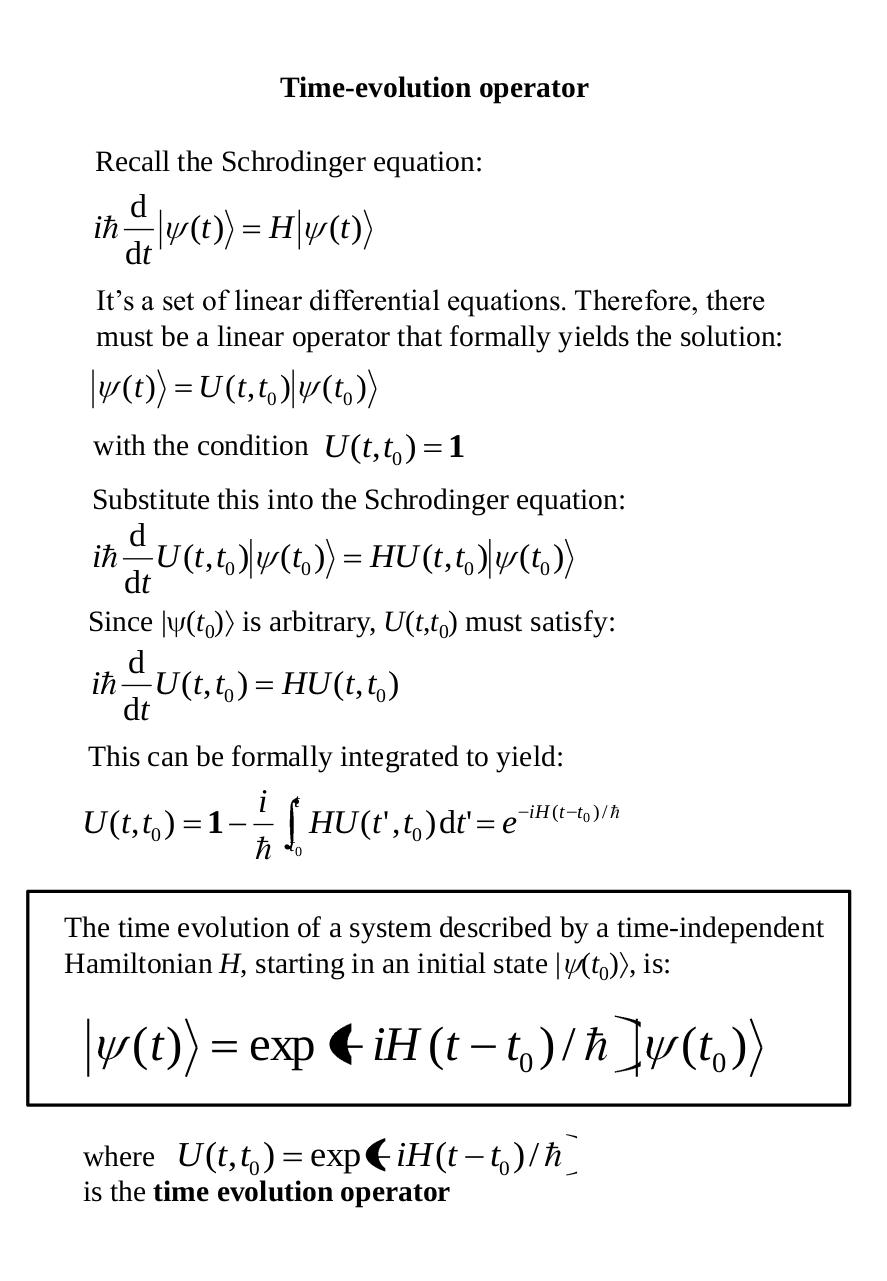# ELEC9705 lecture 05 Operators, Coupling, Entaglement.pdfPage 1 2 34515

#### Text preview

Time-evolution operator
Recall the Schrodinger equation:

i

d
dt

(t )

H

(t )

It’s a set of linear differential equations. Therefore, there
must be a linear operator that formally yields the solution:

(t )

U ( t , t 0 ) ( t0 )

with the condition U (t, t0 )

1

Substitute this into the Schrodinger equation:

i

d
U (t, t0 ) (t0 )
dt

HU (t, t0 ) (t0 )

Since | (t0) is arbitrary, U(t,t0) must satisfy:

i

d
U (t, t0 )
dt

HU (t, t0 )

This can be formally integrated to yield:

U (t, t0 ) 1

i

t
t0

HU (t ' , t0 ) dt ' e

iH ( t t0 ) / 

The time evolution of a system described by a time-independent
Hamiltonian H, starting in an initial state | (t0) , is:

(t )

exp

iH (t t0 ) / 

where U (t, t0 ) exp iH (t
is the time evolution operator

t0 ) / 

(t0 )